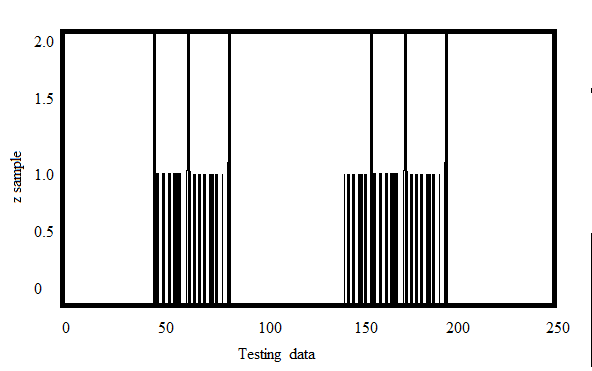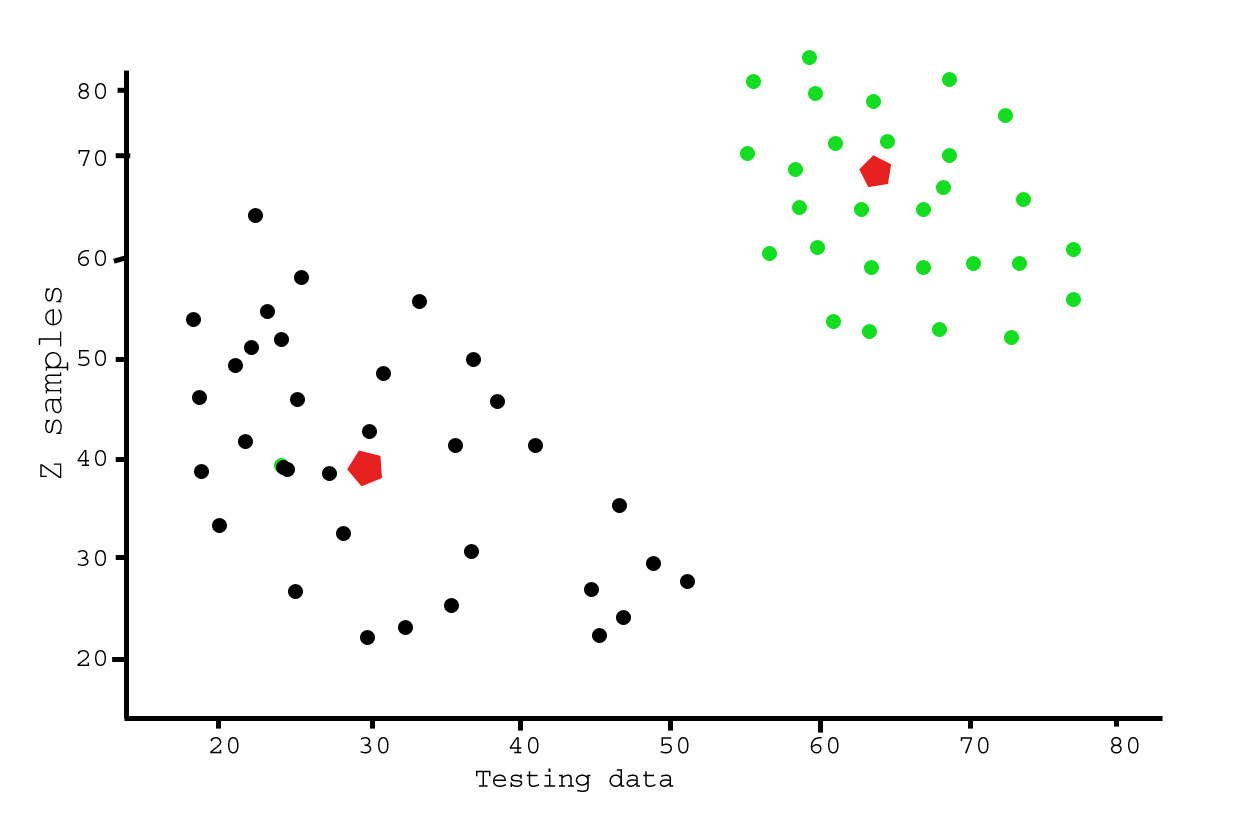# Analysis of test data using K-Means Clustering in Python

This article demonstrates an illustration of K-means clustering on a sample random data using open-cv library.

Pre-requisites: Numpy, OpenCV, matplot-lib
Let’s first visualize test data with Multiple Features using matplot-lib tool.

 `# importing required tools ` `import` `numpy as np ` `from` `matplotlib ``import` `pyplot as plt ` ` `  `# creating two test data ` `X ``=` `np.random.randint(``10``,``35``,(``25``,``2``)) ` `Y ``=` `np.random.randint(``55``,``70``,(``25``,``2``)) ` `Z ``=` `np.vstack((X,Y)) ` `Z ``=` `Z.reshape((``50``,``2``)) ` ` `  `# convert to np.float32 ` `Z ``=` `np.float32(Z) ` ` `  `plt.xlabel(``'Test Data'``) ` `plt.ylabel(``'Z samples'``) ` ` `  `plt.hist(Z,``256``,[``0``,``256``]) ` ` `  `plt.show() `

Here ‘Z’ is an array of size 100, and values ranging from 0 to 255. Now, reshaped ‘z’ to a column vector. It will be more useful when more than one features are present. Then change the data to np.float32 type.

Output:Now, apply the k-Means clustering algorithm to the same example as in the above test data and see its behavior.
Steps Involved:
1) First we need to set a test data.
2) Define criteria and apply kmeans().
3) Now separate the data.
4) Finally Plot the data.

 `import` `numpy as np ` `import` `cv2 ` `from` `matplotlib ``import` `pyplot as plt ` ` `  `X ``=` `np.random.randint(``10``,``45``,(``25``,``2``)) ` `Y ``=` `np.random.randint(``55``,``70``,(``25``,``2``)) ` `Z ``=` `np.vstack((X,Y)) ` ` `  `# convert to np.float32 ` `Z ``=` `np.float32(Z) ` ` `  `# define criteria and apply kmeans() ` `criteria ``=` `(cv2.TERM_CRITERIA_EPS ``+` `cv2.TERM_CRITERIA_MAX_ITER, ``10``, ``1.0``) ` `ret,label,center ``=` `cv2.kmeans(Z,``2``,``None``,criteria,``10``,cv2.KMEANS_RANDOM_CENTERS) ` ` `  `# Now separate the data ` `A ``=` `Z[label.ravel()``=``=``0``] ` `B ``=` `Z[label.ravel()``=``=``1``] ` ` `  `# Plot the data ` `plt.scatter(A[:,``0``],A[:,``1``]) ` `plt.scatter(B[:,``0``],B[:,``1``],c ``=` `'r'``) ` `plt.scatter(center[:,``0``],center[:,``1``],s ``=` `80``,c ``=` `'y'``, marker ``=` `'s'``) ` `plt.xlabel(``'Test Data'``),plt.ylabel(``'Z samples'``) ` `plt.show() `

Output:This example is meant to illustrate where k-means will produce intuitively possible clusters.

Applications:
1) Identifying Cancerous Data.
2) Prediction of Students’ Academic Performance.
3) Drug Activity Prediction.

My Personal Notes arrow_drop_upCheck out this Author's contributed articles.

If you like GeeksforGeeks and would like to contribute, you can also write an article using contribute.geeksforgeeks.org or mail your article to contribute@geeksforgeeks.org. See your article appearing on the GeeksforGeeks main page and help other Geeks.

Please Improve this article if you find anything incorrect by clicking on the "Improve Article" button below.